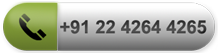• Land Measurement
•1 Sq.Yard = 9 Sq.Feet = 0.8361 Sq.Meter
•1 Sq.Meter = 10.76 Sq.Ft = 1.1960Sq.Yard
•100 Sq.Yds = 900 Sq.Ft = 83 Sq.Meter
•100 Sq.Mtrs = 1076 Sq.Ft = 120 Sq.Yrd
•1 Acre = 4840 Sq.Yds. : 1 Guntha = 121 Sq. Yds.
•1 Acre = 4047 Sq.Mtrs. : 1 Guntha = 101.2 Sq. Mtrs.
•1 Acre = 43560 Sq.Ft. : 1 Guntha = 1089 Sq.Ft.
•1 Acre = 40 Guntha.
•1 Hector = 100 Guntha = 2.471 Acre
•1 Hector = 10000 Sq.Mtrs.